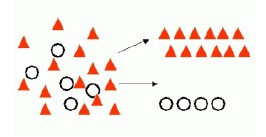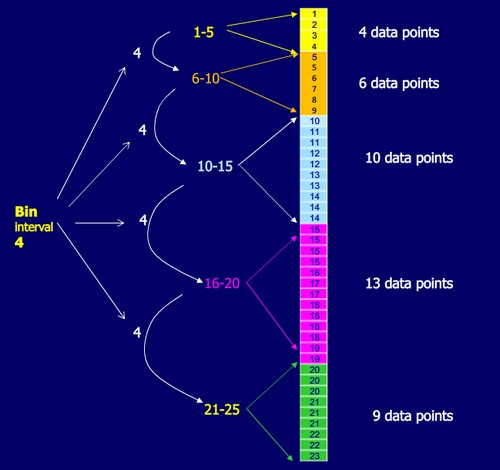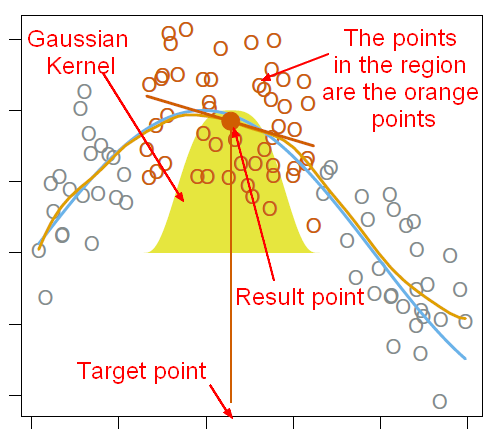# Machine Learning - K-Nearest Neighbors (KNN) algorithm - Instance based learning

“Nearest‐neighbor” learning is also known as “Instance‐based” learning.

K-Nearest Neighbors, or KNN, is a family of simple:

based on Similarity (Distance) calculation between instances.

Nearest Neighbor implements rote learning. It's based on a local average calculation.

It's a smoother algorithm.

Some experts have written that k-nearest neighbours do the best about one third of the time. It's so simple that, in the game of doing classification, you always want to have it in your toolbox.

If you use the nearest neighbor algorithm, take into account the fact that points near the boundary have fewer neighbors because some neighbors may be outside the boundary. You need to correct this bias.

## Type of Model

### Regression

#### Mean

Of all Y value for a X.

#### Neighbourhood

When they are too few data points for a X value in order to calculate the mean, the neighbourhood can be used.

Nearest neighbour averaging can be pretty good for a small number of variable (See accurate ) and large N (in order to get enough point to calculate the average).

## Decision boundary

The nearest neighbor methode produces a linear decision boundary. It's a little bit more complicate as it produces a piece-wise linear decision of the decision boundary with sometimes a bunch of little linear pieces.

The perpendicular bisector of the line that joins the two closest points:

## Remedies against

### All attributes are equally important

Knn assumes that all attributes are equally important. Remedy: attribute selection or weights

### Noisy instances

Noisy instance are instance with a bad target class.

If the dataset is noisy , then by accident we might find an incorrectly classified training instance as the nearest one to our test instance.

You can guard against that by using:

• Majority vote over K nearest neighbours instances (k might be 3 or 4). You look for the 3 or 5 nearest neighbours and choose the majority class amongst those when classifying an unknown point. See k_parameter
• Weight instances according to prediction accuracy
• Identification of reliable “prototypes” for each class

## K Parameter

The K parameter is the first method to guard this method against noisy dataset.

### Best k value

An obvious issue with k nearest neighbour is how to choose a suitable value for the number of nearest neighbours used.

• If it's too small, the method is susceptible to noise in the data.
• If it's too large, the decision is smeared out, covering too great an area of instance space

weka uses cross-validation to select the best value.

The X axis shows the formula 1/K because since K is the number of neighbours, 1/k ⇐ 1.

### With a K close to the size of the whole data set

If we set k to be an extreme value, close to the size of the whole data set, then we're taking the distance of the test instance to all of points in the dataset and averaging those which will probably gives us something close to the baseline accuracy.

### Curse of dimensionality

There is a theoretical guarantee that with a huge dataset and large values of k, you're going to get good results from nearest neighbour learning.

Nearest neighbourhood methods can be lousy when p (the number of variable) is large because of the curse of dimensionality. In high dimension, it's really difficult to stay local.

### Slow

slow as you need to scan entire training data to make each prediction.

## How to

### Discover if the dataset is noisy

By changing the k data set if the accuracy changes a lot between 1 and 5 k it's may be a noisy data set If the data set is noisy, the accuracy figures improves as k got little bit larger but then it would be starting to decrease again.

## Implementation

### Weka

In weka it's called IBk (instance-bases learning with parameter k) and it's in the lazy class folder. KNN is the K parameter. IBk's KNN parameter specifies the number of nearest neighbors to use when classifying a test instance, and the outcome is determined by majority vote.

Weka's IBk implementation has the “cross-validation” option that can help by choosing the best value automatically Weka uses cross-validation to select the best value for KNN (which is the same as k).

## Documentation / Related

Recommended PagesData Mining - (Classifier|Classification Function)

A classifier is a Supervised function (machine learning tool) where the learned (target) attribute is categorical (“nominal”) in order to classify. It is used after the learning process to classify...Data Mining - Decision boundary Visualization

Classifiers create boundaries in instance space. Different classifiers have different biases. You can explore them by visualizing the classification boundaries. Logistic Regression method produces...Machine Learning - Rote Classifier

The “rote” classifier classifies data items based on exact matches to the training set. Otherwise, it search in the training set for one that’s “most like” it. The key concept here is the description...Machine learning - Bootstrap aggregating (bagging)

Bootstrap aggregating (bagging) is a machine learning ensemble meta-algorithm designed to improve the stability and accuracy of machine learning algorithms used in statistical classification and regression....R - K-Nearest Neighbors (KNN) Analysis

in R. where: k is number of neighbours to be considered. train is the training set c1 is the factor of the training set with the true target test is the test set The knn function is...Statistics - (Discretizing|binning) (bin)

Discretization is the process of transforming numeric variables into nominal variables called bin. The created variables are nominal but are ordered (which is a concept that you will not find in true...Statistics - LOcal (Weighted) regrESSion (LOESS|LOWESS)

Popular family of methods called local regression that helps fitting non-linear functions just focusing locally on the data. LOESS and LOWESS (locally weighted scatterplot smoothing) are two strongly...Statistics - Regression

Regression is a statistical analysis used: to predict scores on an numeric outcome variable, based on scores of: one predictor variable: simple regression or multiple predictor variables: multiple...